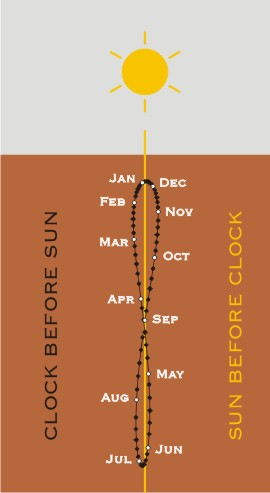# Time>Measurement of time>The equation of time

## How the analemma represents the equation of timeThe figure-of-eight analemma is the equation of time plotted onto a sundial.

The equation of time is the adjustment between solar time and mean time. Solar time runs at a varying speed through the year, because of how the earth orbits the sun. Mean time is the average of how solar time varies and provides a consistent time for clocks. The analemma is the name for a chart of the equation of time.

Earth’s orbit is an ellipse. The orbit runs slightly faster when the earth is nearer to the sun from September to March. Earth’s axis is also tilted. The northern hemisphere tilts towards the sun from March to September.

The combined effects of the ellipse and the tilt result in the length of each solar day being slightly different from the previous day. This is why solar time varies through the year. In November solar time is 16 minutes ahead of mean time, in February it is 14 minutes behind, and on 15 April and 1 September the two times cross over, carrying on in an endless cycle. The analemma represents this endless cycle and is charted on a sundial to let it read like a clock in mean time. It looks like a figure-of-eight loop.